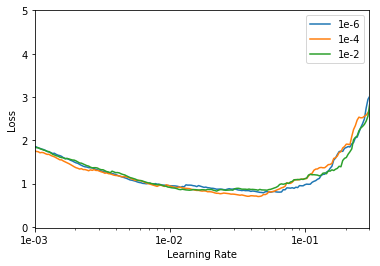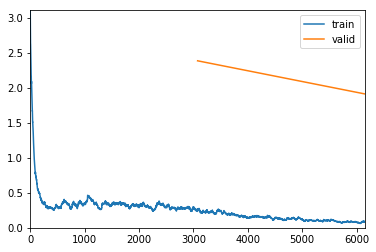# High Error, but High Accuracy

Hi, I’ve encountered a mysterious problem where the validation loss is >1, but reaches 97.5% accuracy. I am working with medical signal data. Here is what I’ve done.

This is the structure of my data:

``````data.info()
0    90589
4     8039
2     7236
1     2779
3      803
Name: label, dtype: int64
``````

To create the dataloader I transformed the medical data to images through Gramian Angular Field transforms. Here is the code for the data loader and learner.

``````class PixelList(ImageList):
def open(self, index):
regex = re.compile(r'\d+')
fn = re.findall(regex, index)
df_fn = self.inner_df[self.inner_df.index.values == int(fn)]
img_pixel = np.squeeze(df_fn.drop(labels=['label','index'],axis=1).values)
#print(int(fn)) Errorchecking
#print(img_pixel.shape) Errorchecking
img_pixel = proc_tfm_vec(img_pixel, augmentations = True)
return vision.Image(pil2tensor(img_pixel, np.float32))

src = (PixelList.from_df(data,'.',cols='index')
.split_by_rand_pct(0.1)
.label_from_df(cols='label')
.transform(tfms=[],size = 224))
input_data = (src.databunch(bs=32).normalize())
arch = densenet169
MODEL_PATH = str(arch).split()
def getLearner():
return create_cnn(input_data, arch, pretrained=True, path='.',
metrics=error_rate, ps=0.5, callback_fns=ShowGraph)

learner = getLearner()
``````

Then I started selecting for learning rate and weight decay by plotting the rate scheduler.

``````lrs = []
losses = []
wds = []
iter_count = 600

# WEIGHT DECAY = 1e-6
learner.lr_find(wd=1e-6, num_it=iter_count)
lrs.append(learner.recorder.lrs)
losses.append(learner.recorder.losses)
wds.append('1e-6')
learner = getLearner() #reset learner - this gets more consistent starting conditions

# WEIGHT DECAY = 1e-4
learner.lr_find(wd=1e-4, num_it=iter_count)
lrs.append(learner.recorder.lrs)
losses.append(learner.recorder.losses)
wds.append('1e-4')
learner = getLearner() #reset learner - this gets more consistent starting conditions

# WEIGHT DECAY = 1e-2
learner.lr_find(wd=1e-2, num_it=iter_count)
lrs.append(learner.recorder.lrs)
losses.append(learner.recorder.losses)
wds.append('1e-2')
learner = getLearner() #reset learner
``````

Plotting the losses gave me this output:

``````# Plot weight decays
_, ax = plt.subplots(1,1)
min_y = 0
max_y = 5
for i in range(len(losses)):
ax.plot(lrs[i], losses[i])
min_y = min(np.asarray(losses[i]).min(), min_y)
ax.set_ylabel("Loss")
ax.set_xlabel("Learning Rate")
ax.set_xscale('log')
#ax ranges may need some tuning with different model architectures
ax.set_xlim((1e-3,3e-1))
ax.set_ylim((min_y - 0.02,max_y))
ax.legend(wds)
ax.xaxis.set_major_formatter(plt.FormatStrFormatter('%.0e'))
``````And then training the algorithm (using fit_one_cycle) gave me a high validation error, but also a high validation accuracy. Is the accuracy indicated the training accuracy? Should I try to get the validation accuracy down? I don’t think I’ve unfrozen the layers behind the last layer (correct me if I’m wrong), and what next steps should I take for this machine learning model? Thanks

``````max_lr = 3e-2
wd = 1e-4
# 1cycle policy
learner.fit_one_cycle(cyc_len=2, max_lr=max_lr, wd=wd)
``````
epoch train_loss valid_loss error_rate time
0 0.255444 2.387325 0.048794 11:20
1 0.082971 1.913248 0.025493 11:24